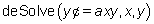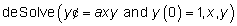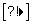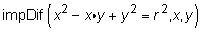##### TI-Nspire For Dummies, 2nd EditionIn the Calculus submenu of the TI-Nspire CAS, the CAS technology allows symbolic manipulation of algebraic expressions as well as numerical calculations just like in the Algebra submenu. The Derivative, Integral, and Limit commands form the cornerstone of the Calculus submenu. Here are some other items contained on the Calculus submenu that are worth mentioning, including a brief description of what they do:

• Tangent Line (& Normal Line): The syntax for this command is tangentLine(Expression, Variable, point). This command returns the equation of the tangent line to the equation y = expression for an x-value equal to point. The Normal Line command works exactly the same way, except that it returns the equation of the line perpendicular to the tangent line at the same point on the curve y = expression.

• Arc Length: The syntax for this command is arclen(Expression, Variable, Start, End). This command gives the arc length of a function (given by Expression) from x = Start to x = End. Try evaluating arclen(f(x), x, a, b) to obtain the textbook formula for the arc length along a curve.

• Differential Equation Solver: The syntax for this command is deSolve(1st or 2nd Degree Differential Equation, Independent Variable, Dependent Variable). The commandreturns the general solution y = ckeaxx, where k is an integer suffix from 1 to 255. The first time you execute this command, TI-Nspire CAS returns the solution y = c1eaxx, where c1 is an arbitrary constant. Subsequent occurrences of this arbitrary constant are denoted c2, c3, and so on. To find a particular solution, include the initial condition(s) with the differential equation. For example, the commandreturns the solution y = eaxx.

Pressand choose the apostrophe to denote a first derivative. Pressand choose the apostrophe again to denote a second derivative.

• Implicit Differentiation: This command gives the implicitly defined derivative of an equation in which one variable is implicitly defined in terms of another variable. The syntax for this command is impDif(Equation, Independent Variable, Dependent Variable). For example, the commandreturns the result (2xy)/(x–2y).

If a term such as xy is in the equation, be sure to separate the variables by including multiplication (r) so that TI-Nspire CAS doesn’t think xy is its own variable.